# Vector Calculus

(redirected from N-dimensional calculus)
The following article is from The Great Soviet Encyclopedia (1979). It might be outdated or ideologically biased.

## Vector Calculus

a mathematical discipline that studies the properties of operations on vectors of Euclidean space. Here, the concept of a vector constitutes the mathematical abstraction of quantities that are characterized not only by a numerical value but also by a direction (for example, force, acceleration, velocity).

Origin and development The origin of vector calculus is closely linked with the needs of physics and mechanics. Until the 19th century, vectors were represented only by the coordinate method, and operations on vectors were reduced to operations on their coordinates. Only in the middle of the 19th century, by the endeavors of a number of scientists, was a vector calculus created in which operations were carried out directly on vectors, without resorting to their coordinate representations. The fundamentals of vector calculus were laid by the British mathematician W. Hamilton and the German mathematician G. Grassmann in their work on hypercomplex numbers (1844-50). Their ideas were used by the British physicist J. C. Maxwell in his work on electricity and magnetism. The American physicist J. Gibbs gave vector calculus its modern form. Russian scientists made significant contributions to the development of vector calculus. First, one should note the work of M. V. Ostrograd-skii, who proved the fundamental theorem of vector analysis. (Ostrogradskii’s formula). The investigations of the Kazan mathematician A. P. Kotel’nikov of the development of helical calculus were of great value to mechanics and geometry. These investigations were continued by the Soviet mathematicians D. N. Zeiliger and P. A. Shirokov. The book Vector Analysis, written by the Russian mathematician P. O. Somov in 1907, greatly influenced the development of vector calculus.

Vector algebra A vector is a directed line segment, that is, a segment whose beginning (also called the vector’s point of application) and end are indicated. The length of the directed line segment, which represents a vector, is called its length or magnitude. The length of vector a is denoted by |a|. Vectors are called collinear if they lie either on the same line or on parallel lines. Two vectors are said to be equal if they are collinear and have the same length and direction. All zero vectors are considered to be equal. Vectors a and b in Figure 1 are collinear and equal. Vector calculus, deals with free vectors.

An important role in vector algebra is played by linear operations on vectors: adding vectors and multiplying them by real numbers. The sum a + b of vectors a and b is the vector extending from the beginning of vector a to the end of vector b such that the beginning of vector b is joined to the end of vector a (see Figure 2). The derivation of this rule is related to the parallelogram rule of vector addition (see Figure 3), whose source is the experimental fact of the addition of forces (vector magnitudes) according to this rule. The construction of the sum of several vectors is clear from Figure 4. The product α a of vector a and the number α is a vector that is collinear with vector a and has a length |α|· |a| and a direction that coincides with the direction of a when α> 0 and is opposite to that of a when α< 0. Vector -1 · a is the inverse vector of a and is denoted by -a. The operations of vector addition and of multiplication of a vector by a number have the following properties:Figure 1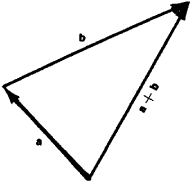Figure 2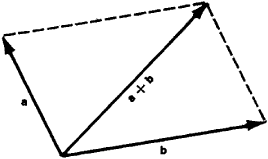Figure 3

(1) a + b = b + a

(2) (a + b) + c = a + (b + c)

(3) a + 0 = a

(4) a + (-a) = 0

(5) 1· a = a

(6) α(βa) = β)a

(7) α(a + b) = αa + αb

(8) (α + β)a = αa + βa

The concept of linearly dependent and linearly independent vectors is often encountered in vector algebra. Vectors a1, a2,…, an are called linearly dependent if there exist such numbers α1, α2,…, αn, of which at least one of them differs from zero, that the linear combination α1a1 + · · · +αnan of these vectors is equal to zero. Vectors a1, a2, … , an that are not linearly dependent are called linearly independent. Let us note that any three nonzero vectors not lying in one plane are linearly independent.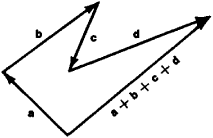Figure 4

The vectors of Euclidean space have the following property: there exist three linearly independent vectors, and any arbitrary four vectors are linearly dependent. This property characterizes the three-dimensionality of the set of vectors under consideration. In conjunction with the properties listed above, the indicated property implies that the set of all vectors of Euclidean space forms a so-called vector space. The linearly independent vectors e1, e2, and e3 form a basis. Any vector a can be uniquely resolved in terms of basis vectors: a = X e1+ Y e2 + Z e3 the coefficients X, Y, and Z are called the coordinates (components) of vector a in the given basis. If vector a has coordinates X, Y, and Z, this can be written as a = {X, Y, Z }.Three mutually orthogonal (perpendicular) vectors, whose lengths are equal to one and which are usually denoted by i, j, and k, form a so-called orthonormalized basis. If these vectors are located with their initial points at one point O, they form a rectangular Cartesian coordinate system in space. The coordinates X, Y, Z of any point M in this system are defined as the coordinates of the vector O M (see Figure 5). The linear operations on vectors, indicated previously, correspond to analogous operations on their coordinates: if the coordinates of vectors a and b are {Xl, Y1, Z1} and X2, Y2, Z2} respectively, then the coordinates of the sum a + b of these vectors are {Xl + X2, Yl + Y2,Z1 + Z2} the coordinates of vector λa are {λX1, λY1, λZ1}.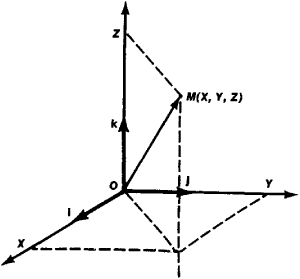Figure 5

The development and application of vector algebra is closely connected with various types of products of vectors: scalar, vector, and mixed. The concept of the scalar product of vectors arises, for example, in examining the work performed by a force F along a given path S: the work is equal to |F| |S| cos φ, where φ is the angle between vectors F and S. Mathematically, the scalar product of vectors a and b is defined as the number denoted by (a, b) and equal to the product of the magnitudes of these vectors and of the cosine of the angle between them:

(a, b) = |a| |bx| cos φ

The quantity |b| cos φ is called the projection of vector b on the axis determined by vector a and is denoted by projab. Therefore, (a, b) = | a | projab. In particular, if a is a unit vector ( |a| = 1), then (a, b) = projab. The following properties of the scalar product are obvious:

(a, b) = (b, a)

(λa,b) = φ(a,b)

(a + b,c) = (a,c) + (b,c)

(a, a) ≥ 0)

where equality with zero occurs only for a = 0. If vectors a and b have the coordinates {X1, Y1, Z1} and {X2, Y2, Z2} respectively, in an orthonormalized basis i, j, k, then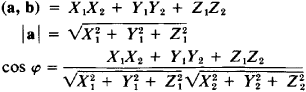The definition of a vector product requires use of the concept of a left- and right-handed ordering of three vectors. The ordered triplet of vectors a, b, c (a is the first vector, b, the second, and c, the third), starting at the same point and not lying in one plane, is called right-handed (left-handed) if the vectors are situated in the same way as the thumb, index, and middle fingers, respectively, of the right (left) hand. Figure 6 shows right-handed (on the right) and left-handed (on the left) triplets of vectors.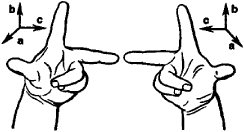Figure 6

The vector product of vectors a and b is the vector denoted by [a, b] and satisfying the following requirements: (1) the length of vector [a, b] is equal to the product of the lengths of vectors a and and of the sine of the angle φ between them (thus, if a and b are collinear, then [a, b] = 0); and (2) if a and b are noncollinear, then [a, b] is perpendicular to both vectors a and b and is directed so that the triplet of vectors a, b, [a, b] is right-handed. The vector product has the following properties:

[a, b] = [b, a]

[(λa), b] = [a, b]

[c, (a + b)] = [c, a] + [c, b]

[a, [b, c]] = b (a, c) - c(a, b)

([a, b], [c, d]) = (a, c )(b, d) - (a, d)(b, c)

If, in an orthonormalized basis i, j, k forming a right-handed triplet, vectors a and b have the coordinates {X1Y1Z1} and {X2Y2Z2}, respectively, then [a, b] = {Y1Z2 - Y2Z1, Z1X2 - Z2X1, X1Y2 - X2Y1}. The concept of vector product is connected with various problems in mechanics and physics. For example, the velocity v of a point M of an object rotating around an axis / with an angular velocity of ω is [ω,r], where r = OM .

The mixed product of vectors a, b, and c is the scalar product of vector [a, b] and vector c: ([a, b], c). It is denoted by abc. The mixed product of vectors a, b, and c that are not parallel to the same plane is numerically equal to the volume of the parallelepiped formed by bringing the vectors a, b, and c to a common initial point; its sign is positive if the triplet a, b, c is right-handed and negative if the triplet is left-handed. If vectors a, b, and c are parallel to the same plane, then abc = 0. The property that abc = bca = cab also holds true. If the coordinates of vectors a, b, and c in an orthonormalized basis i, j, k, which forms a right-handed triplet, are respectively equal to {X1Y1Z1}, {X2Y2Z2},and {X3Y3Z3}, then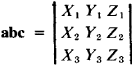Vector functions of scalar arguments In mechanics, physics, and differential geometry frequent use is made of the concept of a vector function of one or several scalar arguments. If a definite vector r is in correspondence to every value of a variable t of a certain set {t } according to a known law, then one says that a vector function r = r(t) is specified by the set {t }. Since vector r is defined by coordinates {x , y , z}, the specification of the vector function r = r(t) is equivalent to the specification of three scalar functions: x = x(t) , y = y(t) , and z = z(t) . The concept of vector function becomes particularly obvious if it is converted to a so-called hodograph of this function, that is, to the locus of the ends of all vectors r(t) joined to the coordinate origin O (see Figure 7). If, in this case, one considers the argument t to be time, then the vector function r(t) represents the law of motion of point M moving along curve L —the hodograph of r(t).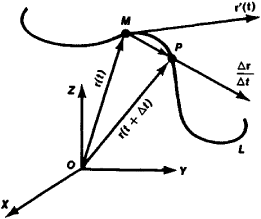Figure 7

The concept of derivative plays an important role in the study of vector functions. This concept is introduced in the following way: to the argument t is added the increment Δt ≠ 0 and the vector Δr = r(t + Δt) - r(t) (the increment Δr is vector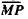in Figure 7) is multiplied by 1/Δt . The limit of the expression Δrt as Δt → 0 is called the derivative of the vector function and is denoted by r(t) or dr/dt . The derivative is the vector that is tangent to the hodograph L at the given point M . If the vector function is regarded as the law of motion of a point along the curve L, then the derivative r’(t) is equal to the velocity of this point’s motion. The rules for computing the derivatives of various products of vector functions are similar to the rules of finding the derivatives of the products of ordinary functions. For example,

(r1, r2)′ = (r1′, r2) + (r1, r2′)

[r1, r2]′ = [r1′, r2] + [r1, r2′]

In differential geometry the vector functions of one argument are used for the definition of curves. Vector functions of two arguments are used for the specification of surfaces.

Vector analysis In mechanics, physics, and geometry the concepts of scalar and vector fields are frequently used. The temperature of a nonuniformly heated plate and the density of a nonhomogeneous body are physical examples of plane and three-dimensional scalar fields, respectively. A vector field is a set of all the velocity vectors of particles of a steady flow of fluid. Other examples of vector fields are the gravitational force field and the electrical and magnetic potentials of an electromagnetic field.

For the mathematical specification of scalar and vector fields, scalar and vector functions are used, respectively. It is clear that the density of an object is a scalar point-function and that the velocity field of the particles of steady liquid flow is a vector point-function. The mathematical apparatus of field theory is usually called vector analysis. For the geometric characterization of a scalar field one uses the concepts of contour lines and equipotential surfaces. The contour line of a plane scalar field is a line on which the function that defines the field has a constant value. The equipotential surface of a spatial field is defined in an analogous way. An example of a contour line is an isotherm—the contour line of the scalar temperature field of a nonuniformly heated plate.

We now consider equipotential surfaces (lines) of a scalar field which pass through a given point M. The maximum change of the function f which defines the field at this point, occurs along a normal to this surface (line) at the point M. This change is characterized by the gradient of the scalar field. The gradient is a vector that is directed along the normal to the equipotential surface (line) at point M in the direction of the increasing ƒ at this point. The magnitude of the gradient is equal to the derivative of ƒ in the indicated direction. The gradient is denoted by the symbol grad ƒ. If the basis is i, j, k, then grad f has the coordinates; for a plane field the gradient coordinates are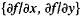. The gradient of a scalar field is a vector field.

A number of concepts are introduced to characterize vector fields: vector lines, vector tubes, circulations of a vector field, and divergence and curl (rotor) of a vector field. In some region Ω, let a vector field be denoted by the vector function a(M) of a variable point M of Ω. A line L in the region Ω is called a vector line if the vector tangent at each of its points M is directed along vector a(M) (see Figure 8).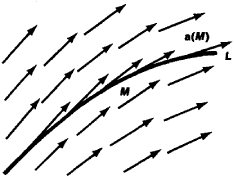Figure 8

If the field a(M) is a velocity field of the particles of a steady flow of a fluid, then the vector lines of this field are the trajectories of the fluid particles. The part of space in Ω that consists of vector lines is called a vector tube (see Figure 9). If one is dealing with the vector field of velocities of the particles of a steady flow of a fluid, then the vector tube is the part of space that a certain fixed volume of fluid “sweeps out” in its motion.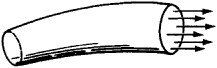Figure 9

Let AB be a smooth curve in Ω, l the length of arc AB measured from point A to the variable point M of this line, and t the unit vector tangent to AB at M . The circulation of the field a(M) along the curve AB is the expression

AB(a,t)dl

If a(M) is a force field, then the circulation of a along AB is the work performed by this field along the path AB.

The divergence of vector field a(M) , which has the coordinates P, Q, R in the basis i, j, k, is defined as the sum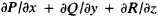and is denoted by the symbol div a. For example, the divergence of the gravitational field created by a certain mass distribution is equal to the density (volumetric) ρ(x, y, z) of this field multiplied by 4π

The curl (or rot) of vector field a(M) is a vector characterizing the “rotational component” of this field. The curl of field a is denoted by rot a or curl a . If P, Q, R are the coordinates of a in the basis i, j, k, then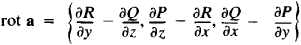Let field a be the velocity field of a fluid flow. We place a small wheel with vanes at a given point of the flow and orient its axis in the direction of rot a at this point. Then the flowrate will be a maximum, and its value will be ½[rot a]. The gradient of a scalar field and the divergence and curl of a vector field are usually called the fundamental differential operations of vector analysis. The following equations, relating these operations, hold true:

div (fa) = (a, grad f) + f div a

rot (fa) = f rot a + [grad f , a]

div [a, b] = (b, rot a) - (a, rot b)

Vector field a(M) is called potential if it is the gradient of some scalar field f(M) . In this case, the field f(M) is called the potential of vector field a. In order that the field a, whose coordinates P, Q, R have continuous partial derivatives, be a potential field, it is necessary and sufficient that the curl of this field vanish. If a potential field is given in a simply connected region Ω, then the potential f(M) of this field can be found from the formula

f = ∫AM (a, t) dl

where AM is any smooth curve connecting a fixed point A of Ω with point M , t is the unit vector tangent to the curve AM, and l is the length of arc AM measured from point A .

Vector field a(M) is called solenoidal or tubular if it is the curl of some field b(M) . Field b(M) is called the vector potential of field a. In order that a be solenoidal, it is necessary and sufficient that the divergence of this field vanish. An important role in vector analysis is played by integral relations: Ostrogradskii’s formula, also designated the fundamental formula of vector analysis, and Stokes’ formula. Let V be a region whose boundary T consists of a finite number of pieces of smooth surfaces and n be the unit vector of the exterior normal to T . Let vector field a(M) be given in the region V such that div a is a continuous function. Then the following holds true:

(1) ∫∫∫v div adv = ∫∫r (a,n)

This is known as Ostrogradskii’s formula.

If a is the velocity field of a steady flow of incompressible fluid, then (a, n) is the volume of fluid that passes through an area on the boundary г in a unit of time. Therefore, the right-hand side of equation (1) is the flow of fluid through the boundary г of body V per unit time. Because, in the case being considered, div a characterizes the intensity of the fluid sources, Ostrogradskii’s formula expresses the following obvious fact: the flow of fluid through a closed surface г is equal to the amount of fluid generated by all the sources inside г. Let a continuous and differentiable vector field a which has a continuous curl rot a be assigned in a region Ω. Let г be an orientable surface consisting of a finite number of pieces of smooth surface, n the unit normal to г, t the unit vector tangent to the edge y of the surface г, and l the length of the arc y . The following relation, called Stokes’ formula, holds true: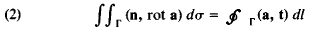Equation (2) expresses the following physical fact: the intensity of the curl of a vector field a through the surface г is equal to the circulation of this field along the curve y . Ostrogradskii’s formula is the source of the invariant (independent of the coordinate system) definition of the fundamental operations of vector analysis. For example, from this formula, it follows that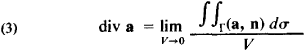Because the expression

∫∫г (a,n)

is the flow of fluid through г and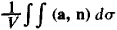is the magnitude of this flow per unit volume, the definition of div a by means of equation (3) indicates that div a characterizes the flux of the source at a given point.

### REFERENCES

Kochin, N. E. Vektornoe ischislenie i nachala tenzornogo ischisleniia, 6th ed. Leningrad-Moscow, 1938.
Dubnov, Ia. S. Osnovy vektornogo ischisleniia, 4th ed. vols. 1-2. Moscow, 1950-52.
Budak, B. M., and S. V. Fomin. Kratnye integraly i riady, 2nd ed. Moscow, 1967.

E. G. POZNIAK